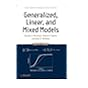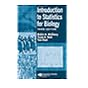Normal view MARC view ISBD view

# Generalized, linear, and mixed models / Charles E. McCulloch, Shayle R. Searle, John M. Neuhaus.

Material type:TextPublisher: Hoboken, N.J. : Wiley, c2008Edition: 2nd ed.Description: xxv, 384 p. : ill. ; 25 cm.ISBN: 9780470073711 (cloth); 0470073713 (cloth).DDC classification: 519.535
Contents:
Table of contents One-way classifications -- Single-predictor regression -- Linear models (LMs) -- Generalized linear models (GLMs) -- Linear mixed models (LMMs) - -Generalized linear mixed models (GLMMs) -- Models for longitudinal data -- Marginal models -- Multivariate models - -Nonlinear models -- Departures from assumptions -- Prediction -- Computing.
Summary: This book provides an up-to-date treatment of the techniques for developing and applying a wide variety of statistical models. It presents unified coverage of the theory behind generalized, linear, and mixed media models and highlights their similarities and differences in various construction, application, and computational aspects. A clear introduction to the basic ideas of fixed effects models, random effects models, and mixed models is maintained throughout, and each chapter illustrates how these models are applicable in a wide array of contexts. In addition, a discussion of general methods for the analysis of such models is presented with an emphasis on the method of maximum likelihood for the estimation of parameters. The authors also provide coverage of the latest statistical models for correlated, non-normally distributed data. This second edition features: a new chapter that covers omitted covariates, incorrect random effects distribution, correlation of covariates and random effects, and variance estimation; a new chapter that treats shared random effected models, latent class models, and properties of models; a revised chapter on longitudinal data, which now includes a discussion of generalized linear models, modern advances in longitudinal data analysis, and the use between and within covariate decompositions; expanded coverage of marginal versus conditional models, and numerous new and updated examples.
Tags from this library: No tags from this library for this title.Average rating: 0.0 (0 votes)
Item type Current location Collection Call number Copy number Status Date due Barcode Item holdsText
Reserve Section
Non-fiction 519.535 MCG 2008 (Browse shelf) C-1 Not For Loan 26683Text
Circulation Section
Non-fiction 519.535 MCG 2008 (Browse shelf) C-2 Available 27044Text
Circulation Section
Non-fiction 519.535 MCG 2008 (Browse shelf) C-3 Available 27045
Total holds: 0
##### Browsing EWU Library Shelves , Shelving location: Circulation Section Close shelf browser519.535 KOI 2014 Introduction to multivariate analysis : 519.535 KOI 2014 Introduction to multivariate analysis : 519.535 LIM 1999 Models for repeated measurements / 519.535 MCG 2008 Generalized, linear, and mixed models / 519.535 MCG 2008 Generalized, linear, and mixed models / 519.535 MCI 2007 Introduction to statistics for biology / 519.535 MCI 2007 Introduction to statistics for biology /

Includes bibliographical references (p. 364-377) and index.

Single-predictor regression --
Linear models (LMs) --
Generalized linear models (GLMs) --
Linear mixed models (LMMs) - -Generalized linear mixed models (GLMMs) --
Models for longitudinal data --
Marginal models --
Multivariate models - -Nonlinear models --
Departures from assumptions --
Prediction --
Computing.

This book provides an up-to-date treatment of the techniques for developing and applying a wide variety of statistical models. It presents unified coverage of the theory behind generalized, linear, and mixed media models and highlights their similarities and differences in various construction, application, and computational aspects. A clear introduction to the basic ideas of fixed effects models, random effects models, and mixed models is maintained throughout, and each chapter illustrates how these models are applicable in a wide array of contexts. In addition, a discussion of general methods for the analysis of such models is presented with an emphasis on the method of maximum likelihood for the estimation of parameters. The authors also provide coverage of the latest statistical models for correlated, non-normally distributed data. This second edition features: a new chapter that covers omitted covariates, incorrect random effects distribution, correlation of covariates and random effects, and variance estimation; a new chapter that treats shared random effected models, latent class models, and properties of models; a revised chapter on longitudinal data, which now includes a discussion of generalized linear models, modern advances in longitudinal data analysis, and the use between and within covariate decompositions; expanded coverage of marginal versus conditional models, and numerous new and updated examples.

Applied Statistics

There are no comments for this item.